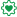# New to Qlik Sense

Discussion board where members can get started with Qlik Sense.

cancel
Showing results for
Did you mean:
HighlightedCreator III

## Aggregate issue

sum({<Is = {'N'},IsH = {'N'},[Date]= {">=\$(vFrom)<=\$(vTo)"},
[order Date]= {">=\$(vGFrom)<=\$(vGTo)"}> }[Cost])

I used Aggregate as below but getting error. i want to use aggr function because the total when exported in excel was not correct . Am i missing something from bellow expression

sum(aggr({<Is = {'N'},IsH = {'N'},[Date]= {">=\$(vFrom)<=\$(vTo)"},
[order Date]= {">=\$(vGFrom)<=\$(vGTo)"}> }[Cost]))

Labels (1)
• ### AGGR()

2 Replies
HighlightedEmployee

## Re: Aggregate issue

The aggr() function needs a field to aggregate by, this is missing in your example

HighlightedCreator III

field is cost